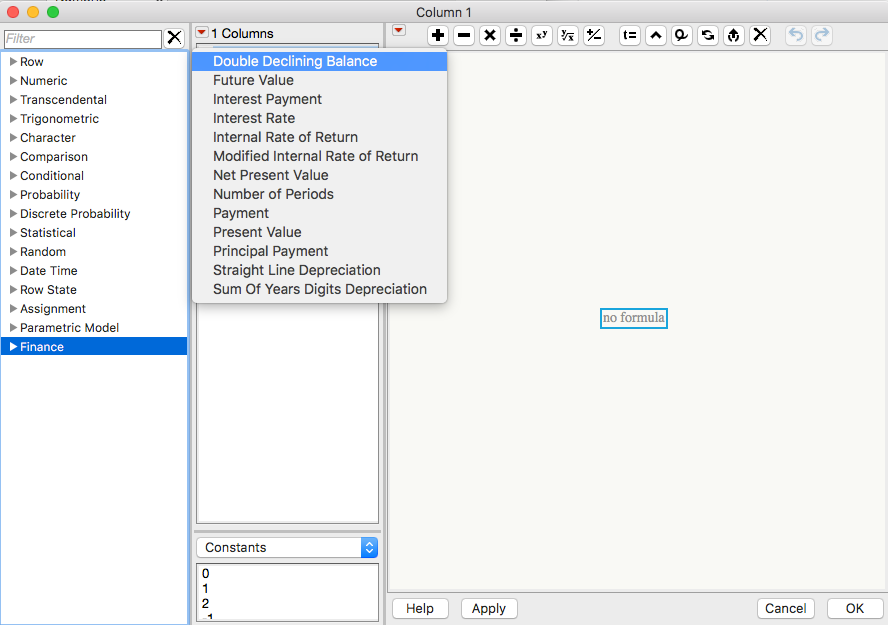Our World Statistics Day conversations have been a great reminder of how much statistics can inform our lives. Do you have an example of how statistics has made a difference in your life? Share your story with the Community!
Choose Language Hide Translation Bar
Highlighted

## Formula extraction for loan

Hello,

I want to extract from a loan schedule the formula that represents the sum of cash interest interests over the loan period and the formula that corresponds to principal repayment knowing that there is also compounded interest. I want to define a variable to change while others are predefined (example interest rate is defined but the variable is the monthly repayment of the loan which I can define as x1, x2, etc.)

My question is:

1- how to input a loan schedule in JMP Pro

2- how to instruct JMP Pro to display the formula for the sum mentioned above

4 REPLIES 4
Highlighted

## Re: Formula extraction for loan

To get started, I would review JMP's financial functions (shown below in the Formula Editor):Highlighted

## Re: Formula extraction for loan

Thanks, but how can I build a loan schedule and how I can extract the formula that I mentioned? Please clarify as I need to figure it out urgently today. Thanks

Highlighted

## Re: Formula extraction for loan

Unfortunately, I don't know enough about what you are trying to do to help. I suspect that might be true for others too (but you might be lucky). Do you have an example in, for instance, Excel?

Highlighted

## Re: Formula extraction for loan

Hello,

The script below creates a table that has Principal and Interest payment information for a 30 year loan with monthly payments. You can adjust the initial Principal and Interest Rate in the table variables. I also included columns for cumulative principal and interest payments as well as the remaining balance. I'm not sure if this is what you were looking for, but I hope it helps you get there.

Michael

``````New Table( "Loan Payments",
New Table Variable( "Principal", 100000 ),
New Table Variable( "Interest Rate", 0.04 ),
New Column( "Month", Numeric, "Continuous", Format( "Best", 12 ), Set Values( 1 :: 360 ) ),
New Column( "Principal Payment",
Numeric,
"Continuous",
Format( "Currency", "USD", 17, 2 ),
Formula( Principal Payment( :Interest Rate / 12, :Month, 360, -:Principal ) )
),
New Column( "Interest Payment",
Numeric,
"Continuous",
Format( "Currency", "USD", 17, 2 ),
Formula( Interest Payment( :Interest Rate / 12, :Month, 360, -:Principal ) )
),
New Column( "Total Payment",
Numeric,
"Continuous",
Format( "Currency", "USD", 17, 2 ),
Formula( Payment( :Interest Rate / 12, 360, -:Principal ) )
),
New Column( "Cumulative Principal",
Numeric,
"Continuous",
Format( "Currency", "USD", 17, 2 ),
Formula( Col Cumulative Sum( :Principal Payment ) )
),
New Column( "Cumulative Interest",
Numeric,
"Continuous",
Format( "Currency", "USD", 17, 2 ),
Formula( Col Cumulative Sum( :Interest Payment ) )
),
New Column( "Remaining Balance",
Numeric,
"Continuous",
Format( "Currency", "USD", 17, 2 ),
Formula( :Principal - :Cumulative Principal )
)
)``````
Michael Crotty
Sr Statistical Writer
JMP Development
Article Labels

There are no labels assigned to this post.